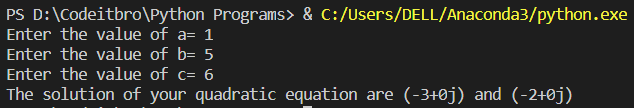# Python 3 Program To Solve A Quadratic Equation

2801

In this tutorial, you will find the Python 3 program to solve a quadratic equation. To understand this example, you should have the basic knowledge of Python programming concepts such as variables, data types, and operators.

If you are looking for some good books to learn Python, then do check out our recommended list

## Python 3 Program To Solve A Quadratic Equation

Formula to calculate a quadratic equation = ax² + bx + c = 0, where a, b and c are real numbers and a ≠ 0. In the Python code below, users will have to enter the values of a, b, and c and then the program will output the solutions of the quadratic equation.

### Source Code

```import cmath # import complex math module
a = float(input("Enter the value of a= "))
b = float(input("Enter the value of b= "))
c = float(input("Enter the value of c= "))
d = (b**2) - (4*a*c) ## calculate the discriminant
sol1 = (-b-cmath.sqrt(d))/(2*a) # Calculating the first solution
sol2 = (-b+cmath.sqrt(d))/(2*a) # Calculating the second solution